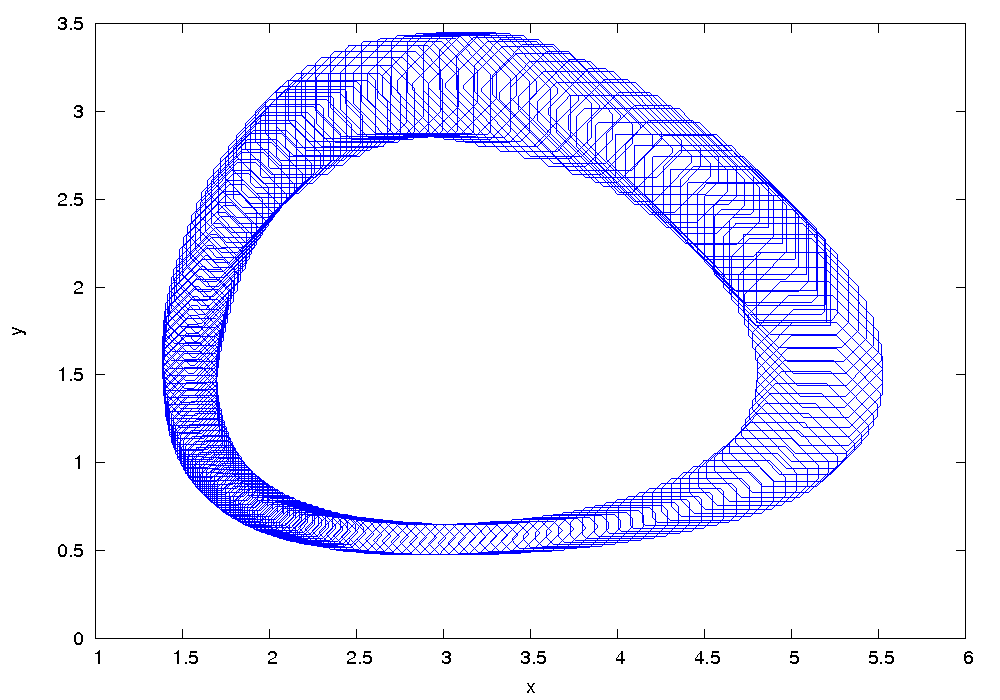## Lotka-Volterra

 Flow* Lotka_Volterra.model

## Model description

The 2-dimensional Lotka-Volterra system depicts the populations change of a class of predators and a class of preys. The growth rate of preys’ population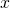over time is given by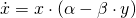wherein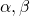are constant parameters and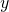is the population of predators. It gives that the number of preys grows exponentially without predation. The population growth of predators is governed by the differential equation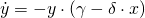whereinare constant parameters. We set those parameters as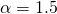,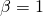,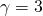and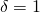.

## Reachability setting

We consider the initial set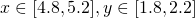.

## Results

The following figure shows an overapproximation computed by Flow* for the time horizon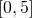: